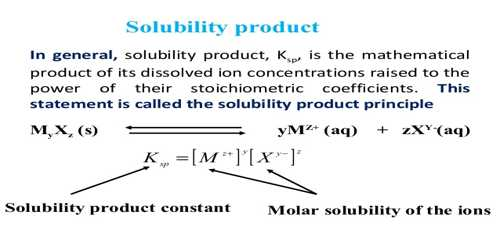Chemistry

# Solubility and Solubility ProductSolubility and Solubility Product

Solubility is defined as the maximum amount of solute that can be dissolved in a solvent at equilibrium. The solubility product constant (K) describes the equilibrium between a solid and its constituent ions in a solution. When an ionic compound dissolves in water the ions get separated. If one keeps on adding more of the solute a situation is reached when no more solute can be dissolved. At that position, the solution is said to be saturated. A saturated solution in contact with solid solute constitutes an equilibrium system. This has been, shown to be a dynamic equilibrium – ions from the solution being deposited as solid solute and solid solute moving to the solution in the form of separated ions, both proceeding at the same rate so that there is no change in concentration. The equilibrium can be shown as,

Solute (in solution) ↔ Solute (solid)

The concentration of the saturated solution is its solubility. This is expressed as g L-1 or mol-1. As long as the temperature is constant and no other compound is added the concentration of the solution remains constant.When a sparingly soluble solute, say BaSO4, dissolved in water the amount of solute in the solution is very little and the following equilibrium is established.

BaSO4 (s) + aq ↔ Ba2+ (aq) + SO42- (aq)

In calculating the solubility products the concentrations of ions are expressed in mol L-1. Like any equilibrium constant solubility product, Ksp, depends on the temperature and remains constant even if other salts are added to the solution. The solubility products of some compounds are given in Table.

Table: Solubility product of some compounds at 25°C